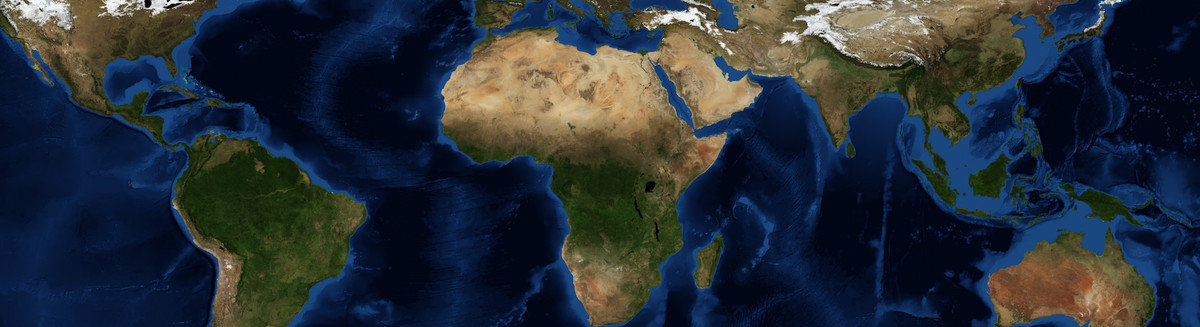This is a Preprint and has not been peer reviewed. This is version 1 of this Preprint.

##### Authors

Mathieu Daëron, Pieter Vermeesch

##### Abstract

Least-squares regression methods are mathematically powerful, conceptually and computationally simple, and widely used in many fields. However, none of the commonly-used flavors of least-squares regression, such as York regression or Generalized Least Squares (GLS), take into account the full set of covariances between all observed (x,y) values. Here we describe the Omnivariant Generalized Least Squares (OGLS) method to fit a model of the form y=f(x), accounting for the full error correlation structure of the (x,y) data, based on a first-order linear propagation of the uncertainties in all variables into errors in y residuals, followed by minimizing the vector of y residuals with respect to the Mahalanobis norm defined by its covariance matrix. This approach may be described as a generalization of both York regression and GLS. It is mathematically exact for straight-line fits, and is also suitable for many non-linear models. Here we describe the principles of OGLS regression and discuss its properties, caveats, and practical use, and provide two consistent open-source implementations in Python and R. To illustrate how various fields of geochronology and stable-isotope geochemistry may benefit from this new method, we discuss how OGLS may specifically apply to 40Ar/39Ar dating and how it provides robust mathematical evidence that Δ47 carbonate calibrations in the recently defined I-CDES metrological scale are statistically indistinguishable, effectively solving long-standing methodological discrepancies.

##### DOI

https://doi.org/10.31223/X5K394

##### Subjects

Applied Mathematics, Earth Sciences, Geochemistry

##### Keywords

statistics, least squares, Geochronology, clumped isotopes

##### Dates

Published: 2023-09-22 05:11

Last Updated: 2023-09-22 09:11